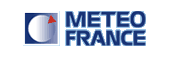Estimation of DFS for each observation type Degree of Freedom SignalGRAPHS DISPLAY :
observation number observation number of non satellite data observation number of satellite data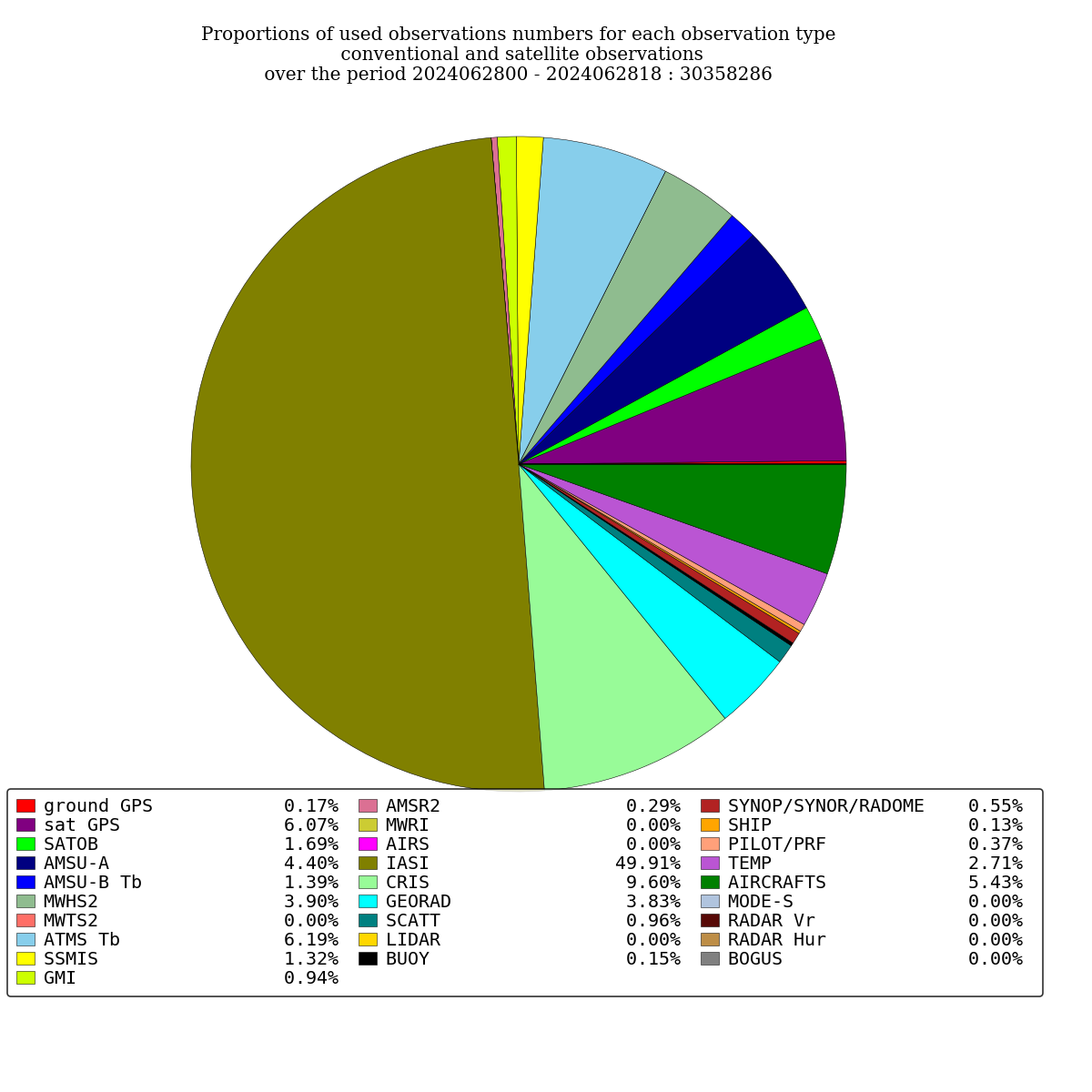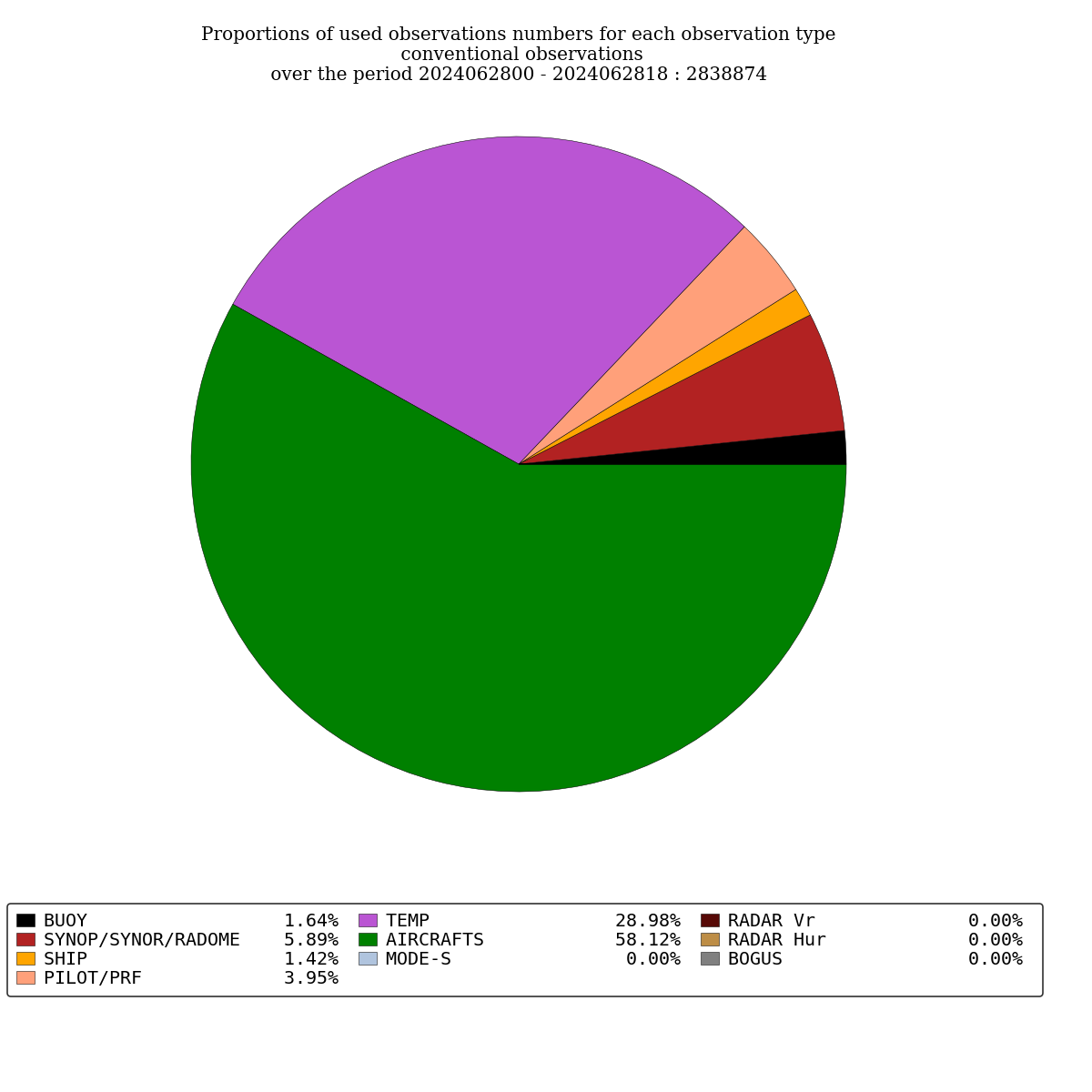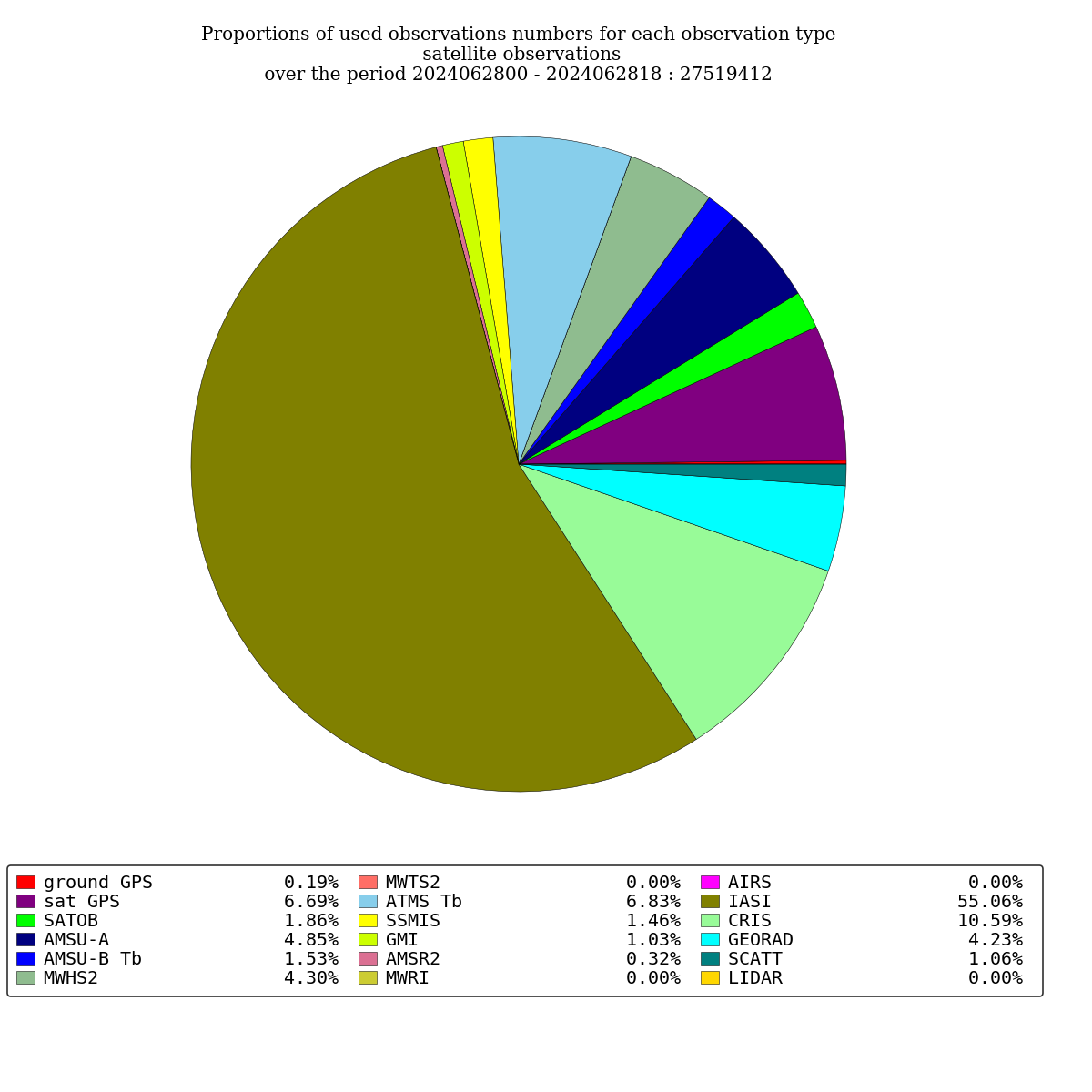DFS DFS of non satellite data DFS of satellite data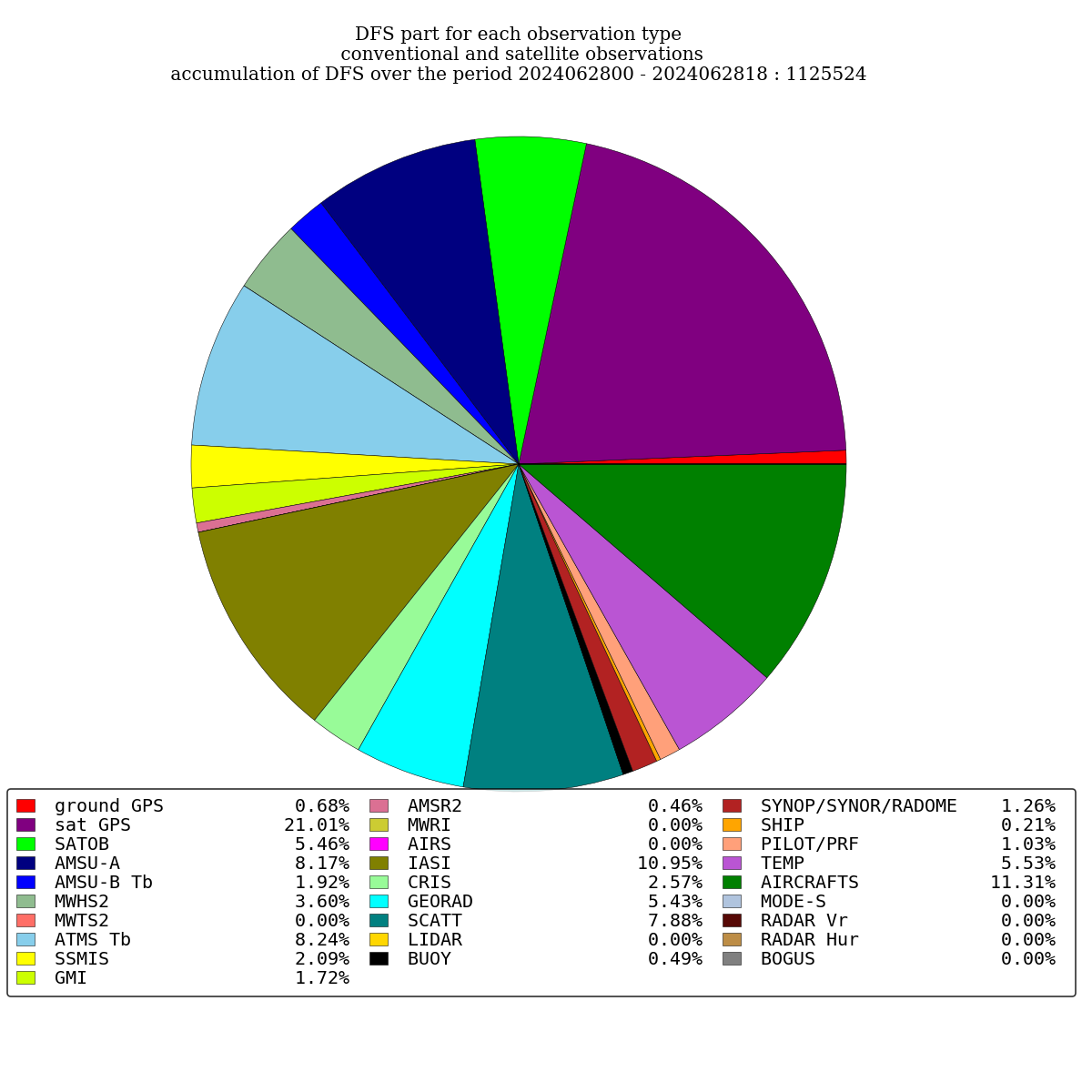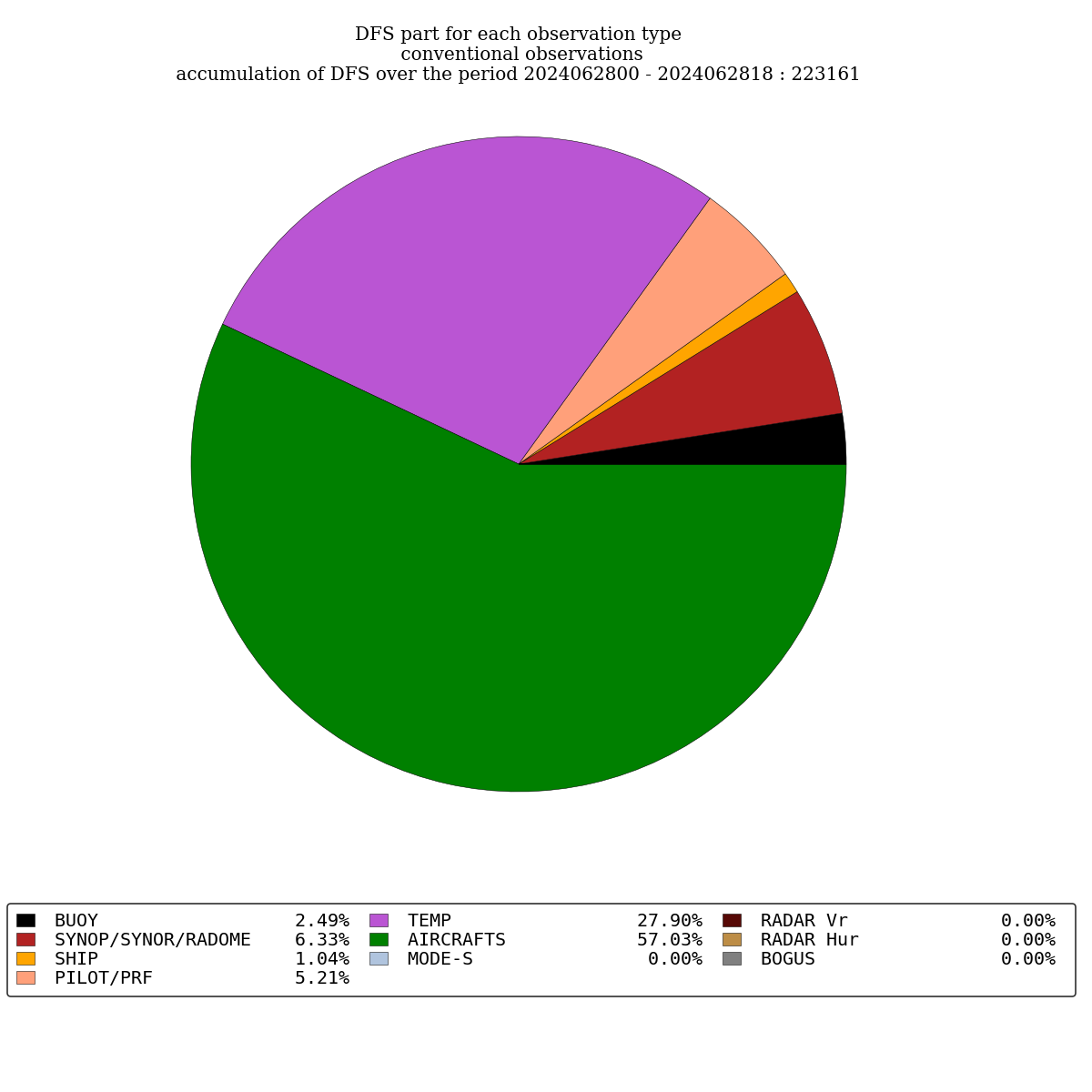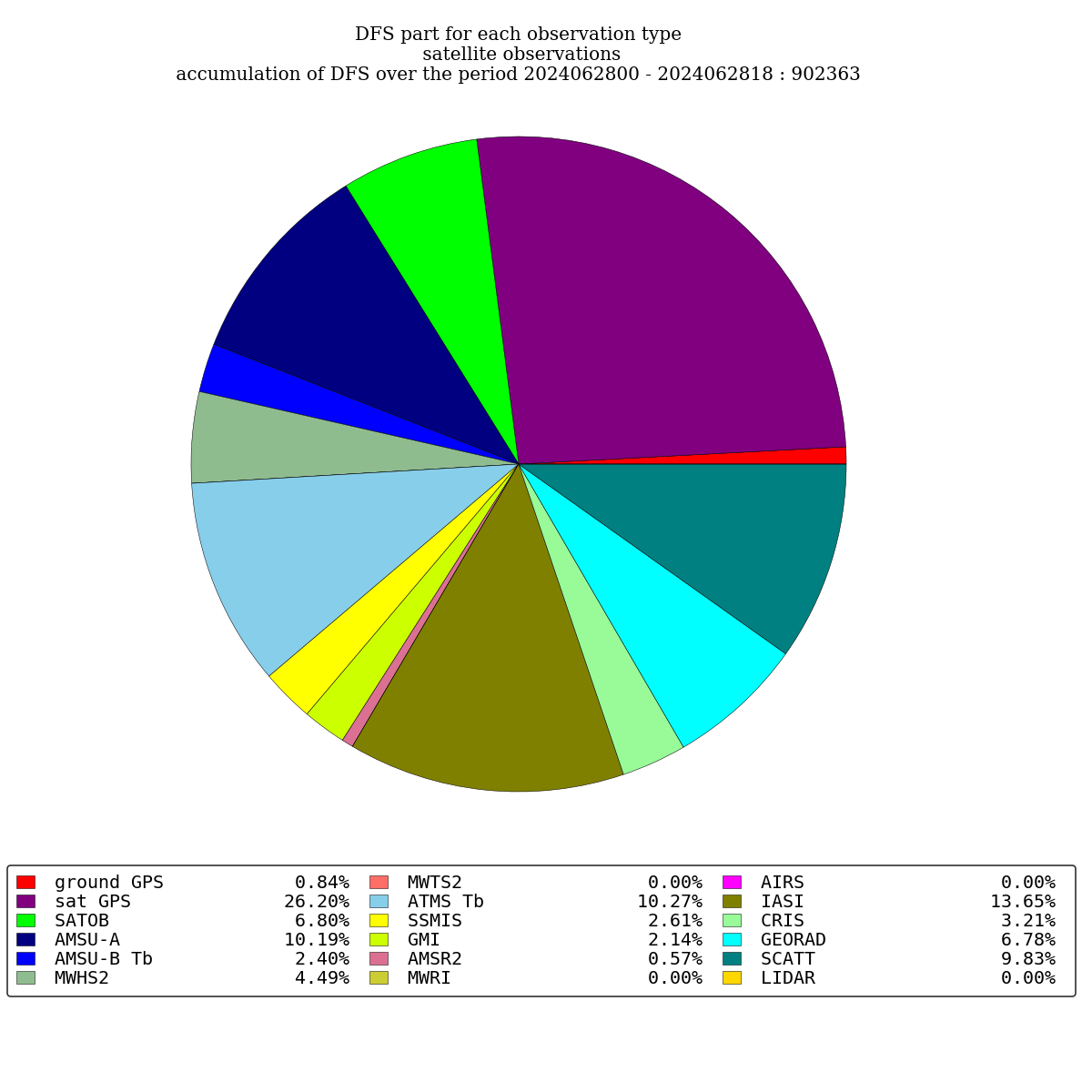We consider here one day of 24 hours assimilation system in order to compute, for each observation type categories, percent parts of : number of used observations for each analysis DFS Degree of Freedom Signal : quantify the power of an observation type to modify assimilation system Numbers of used observations as well as DFS are cumulated over each analysis network of a considered 24 hours assimilation system. Finally, the method used here in order to compute DFS is the one which applies the disrupted screening process. DFS values do not really change in time so DFS graphs computed over 24 hours of operational NWP assimilation system are representative of averaged significance into analysis of each observation type. DFS plots are updated at each important update of our operational NWP assimilation system : in practice, it means when NWP e-suites become operational.